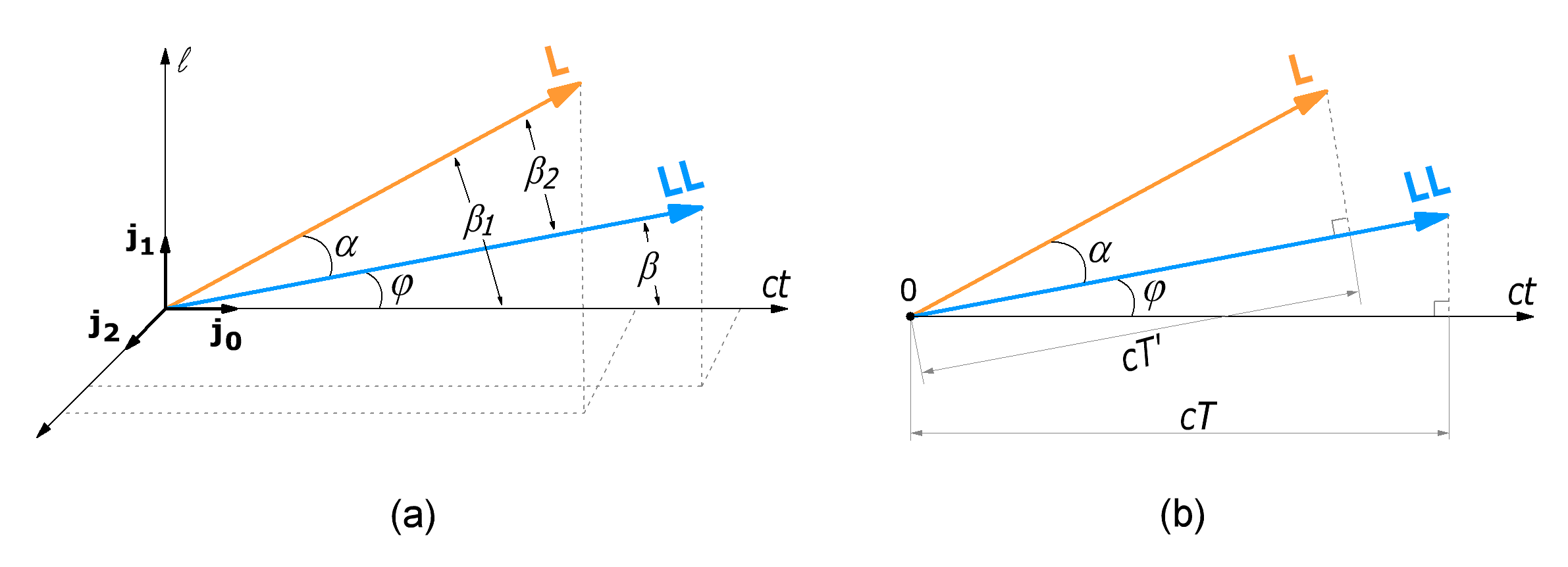Research website of Vyacheslav Gorchilin
2019-10-23
The Doppler Effect. Geometry and mathematics
In this article, with the help of two global vectors of length one, we show a simple derivation of the formula for relavistic Doppler effect . As before, there will not be differentsirovaniya and the inertial reference system, all to be built on a conventional vector algebra, and the effect will be obvious from geometric constructions.
Let us formulate the task. Relative to a fixed coordinate system (reference basis, where the receiver is located) is moving the point source of oscillations of a certain frequency (the transmitter) to the receiver. You want to find the frequency offset in the receiver relative to the frequency emitted by the transmitter.
We will go through consideration of the geometry of two global vectors of length, the first of which $$\mathbf{L}$$ represents the motion of a point, and the second $$\mathbf{LL}$$ — shows the movement of the waves, which geometrically also we will present in the form of another point, moving relative to the first. This approach will demonstrate the simplicity of the method and to even do without vector algebra, as we will take into account the already known formulas previously derived.

The term "global vector of length" next, we shall briefly denote as: GVL. Do not forget that in the real space it is multidimensional and reflect it in a figure we can only partially.

An orthonormal basis $$\mathbf{j_0\ldots j_n}$$ — will call support, because about him we will calculate the Doppler shift. Suppose that relative to the reference basis uniformly moving point with a certain speed $$v_1$$ and the vector is $$\mathbf{L}$$ in (1) is displayed in orange. Regarding the first point, and its base, moving the second — also known speed $$v_2$$. Global vector $$\mathbf{LL}$$ will form with respect to the first GVL angle $$\alpha$$, and the reference basis is the angle $$\varphi$$. Figure (1) this GVL painted blue. We are left with an unknown velocity $$v$$, which is measured is measured between $$\mathbf{LL}$$ and the reference basis is the relativistic difference of the two velocities: $$v_1$$ and $$v_2$$.

Recall that each speed corresponds to its relative value, for example: $$\beta = v/c$$, $$\beta_1 = v_1/c$$ $$\beta_2 = v_2/c$$, where $$c$$ is the speed of light.Fig.1. Two GVL corresponding to the two scalar speeds. Right (b) shows a simplified but more visual version of these vectors and segments, the ratio of which you must find
To solve this problem we just need to find the ratio of two line segments of the vectors (figure 1b): $D = {cT \over cT'} = {T \over T'} \qquad (1.1)$ But to find them let's look first at the reflection of these two vectors on the time axis. From figure (1b) immediately shows that: $cT = |\mathbf{LL}| \cos(\varphi), \quad cT' = |\mathbf{L}| \cos(\alpha) \qquad (1.2)$ Now we Express the angles in the figure using the appropriate Lorentz factors $\cos(\varphi) = 1 / \gamma, \quad \cos(\alpha) = 1 / \gamma_2 \qquad (1.3)$ and reducing the speed of light, to obtain a simple relationship: $T = t / \gamma, \quad T' = t / \gamma_2 \qquad (1.4)$

As a reminder. In these formulas, the corresponding Lorentz Factors are defined as follows: $$\quad \gamma = 1 / \sqrt{1 - \beta^2},\, \gamma_1 = 1 / \sqrt{1 - \beta_1^2},\, \gamma_2 = 1 / \sqrt{1 - \beta_2^2}$$ and the module (length) GVL — so: $$|\mathbf{L}| = |\mathbf{LL}| = ct |\mathbf{R}| = ct$$.

Previously we mentioned about this apparent absolute geometric reduction of time in moving frames. This property is now we need to find its relative value. So, from the previous relations it follows: $D = {T \over T'} = {\cos(\varphi) \over \cos(\alpha)} = {\gamma_2 \over \gamma} \qquad (1.5)$ Where it remains to find $$\gamma$$ and the corresponding relative speed $$\beta$$. Such proof we have already given for the amount of the difference of the velocities: $\beta = {\beta_1 - \beta_2 \over 1 - \beta_1 \beta_2} \qquad (1.6)$ By the way, the relativistic Doppler effect is directly associated with the composition of velocities, and it, in turn, a slowing of time in moving frames. The latter is a consequence of the global law of conservation of energy and its redistribution.
Inserting (1.6) under the square root, we can now find the Lorentz factor of the difference of the velocities: $\gamma = 1 / \sqrt{1 - \beta^2} = \gamma_1 \gamma_2 (1 - \beta_1 \beta_2) \qquad (1.7)$ If we now substitute this expression in (1.5), we get: $D = {1 \over \gamma_1 (1 - \beta_1 \beta_2)} \qquad (1.8)$ In the most General form of the vector $$\mathbf{LL}$$ can optionally be rotated relative to $$\mathbf{L}$$ by angle $$\theta$$ in the perpendicular plane. Then the formula will be supplemented by the cosine of that angle will get the following final form: $D = {T \over T'} = {1 \over \gamma_1 (1 - \beta_1 \beta_2 \cos(\theta))} \qquad (1.9)$ i.e. in a situation when the transmitter is radially removed from the receiver, as in figure (1), this angle is zero, and if approaching something equal to $$\pi$$. If we suppose that the moving point of the emitted wave with a frequency $$f'$$, we can determine the frequency $$f$$ at the receiver, which is located fixedly relative to the reference basis, so: ${f \over f'} = \gamma_1 (1 - \beta_1 \beta_2 \cos(\theta)) \qquad (1.10)$ Recall that here $$f'$$ is the frequency at which the moving transmitter emits the wave, and $$f$$ — frequency, fixed stationary receiver (relative to the reference basis). The last formula does not correspond to the relativistic Doppler effect , and looks relatively classic like a mirror. But in this form it reveals the essence of some of the effects that previously could not be explained from the point of view of the theory of relativity. About this next section.

The materials used
1. Wikipedia. The Effect Of Doppler.